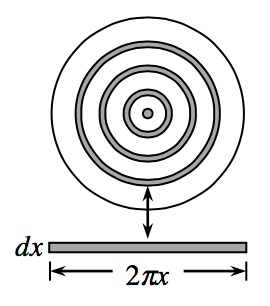### Home > APCALC > Chapter 8 > Lesson 8.1.6 > Problem8-61

8-61.

Instead of using an area formula, Joshua wants to use calculus to find the area of a circle. He realizes that one way to shade a circle is to draw thin concentric circles from the center out, so close together that they entirely cover the circle.

To calculate the area, he needs to add up the area covered by each “circumference” of these circles. If the radius starts at a value of $0$ and ends at a value of $r$, use an integral to prove that the sum of these circumferences equals the area of the circle.Instead of integrating disks, imagine integrating rectangles (or infinitely skinny prisms) that wrap around a center point to create a solid.

$\text{Area of circle }=\int_{0}^{r}(\text{Circumference})dx$

Write an expression for the circumference in terms of $r$.